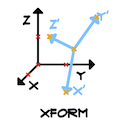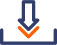## XFORM: the transformation matrix

Did you ever wonder how to use XFORM? Actually it is not that difficult and can come in handy when dealing with planes that are not horizontal or vertical. The EXTRUDEDSHELL, REVOLVEDSHELL, RULEDSHELL commands also use an internal XFORM transformation for defining the orientation of the shell’s cross-section.

#### Syntax

In the GDL reference guide XFORM syntax is written as:

```	XFORM	a11, a12, a13, a14,
a21, a22, a23, a24,
a31, a32, a33, a34```

[two_third] All these numbers define a transformation matrix. The less technical fact behind the matrix is that it contains the desired new coordinate systems’ three axes and origo, expressed in old coordinate system coordinates. The first column of the command defines the endpoint of the new X axis, the second column the new Y axis, the third the new Z, all these relative to the new origo given in the fourth column. [/two_third]
[one_third last=last][/one_third]

```	XFORM	x_axis(x), 	y_axis(x), 	z_axis(x),	origo(x),
x_axis(y), 	y_axis(y), 	z_axis(y), 	origo(y),
x_axis(z), 	y_axis(z),	z_axis(z), 	origo(z)```

That means that if the vectors of the new coordinate system are known, you can simply write those in the matrix. You may have these vectors when they depend on hotspots which the user can freely move in space, or they can be expressed from other parameters. Doing rotations to get to a tilted plane is difficult, involving trigonometric calculations and there are quite a few possibilities to divide by zero.
With XFORM, you have to take care that:

• neither two axes overlap,
• and neither of their length is 0,

such would give errors as “Transformation matrix is singular”, or later during modeling “Not enough edges in generatrix” or “Polygon is degenerated”.

#### Some examples to demonstrate the mechanism of the matrix

`ADD 1, 2, 3` would look like this:

```	XFORM	1,		0,		0,		1,
0,		1,		0,		2,
0,		0,		1,		3```

`MUL 2, 3, 4`:

```	XFORM	2,		0,		0,		0,
0,		3,		0,		0,
0,		0,		4,		0```

```ADD 1, 2, 3 MUL 2, 3, 4```
:

```	XFORM	2,		0,		0,		1,
0,		3,		0,		2,
0,		0,		4,		3```

but order is important!
```MUL 2, 3, 4 ADD 1, 2, 3```:

```	XFORM	2,		0,		0,		2,
0,		3,		0,		6,
0,		0,		4,		12```

`ROTZ 90`:

```	XFORM	0,	       -1,		0,		0,
1,		0,		0,		0,
0,		0,		1,		0```

`ROTZ a`:

```	XFORM	cos(a),		cos(a+90),	0,		0,
sin(a),		sin(a+90),	0,		0,
0,		0,		1,		0```

`ROTZ` and stretch so that U(x, y, 0) will be x axis:

To get the y axis, rotate x by 90°. Rotating by 90° in 2D is simple.

```	XFORM	x,	       -y,		0,		0,
y,		x,		0,		0,
0,		0,		1,		0```

`ROTZ` so that x axis goes through P(x, y, 0):

each axis stays 1 m long, so divide each coordinate by the length of the vector P

```	L = sqr(x ^2 + y ^2)
XFORM	x / L,	       -y / L,		0,		0,
y / L,		x / L,		0,		0,
0,		0,		1,		0```

The new axes don’t have to be necessarily perpendicular, this skewing effect cannot be done with other transformation commands:

```	XFORM	1,		0,		0.2,		1,
0,		1,		0.4,		2,
0,		0,		1,		3```See attached example1 to get a feel how this skewing works. You can move the endpoints of axis X and Y freely in space, the Z axis will stay vertical. A distorted cube of size 1 meter is shown at the origo, each of its sides aligned on the axes.

### Applied vector geometry

A harder case is when perpendicular axes are needed, but you only have three points that define a plane. Some vector geometry calculations will be needed.
Either two of the points can be used as an X axis. You will need a Z that is perpendicular to the plane, and a Y that is on the plane and perpendicular to X.

#### The “vector product” (also called “cross product”) operation

[two_third] It is defined as resulting in a vector that is perpendicular to both of its operands. In other words, it results in the normalvector of the plane that is defined by its two operands. (Two vectors on a common starting point define three points, and three points define a plane.) It can be computed easily: (see proof here)

```	S = U × V
S = U * V - U * V
S = U * V - U * V
S = U * V - U * V```

The up/down direction of S will be such that U, V, S will form a right-handed coordinate system (U the thumb, V the index finger, S the middle finger) [/two_third]

Given any U, V:

• newX = U
• newZ = newX × V
• newY = newZ × newX (newZ and newX are already perpendicular, we need newZ × newX order to get the correct orientation)
• finally probably you will want each axis to have unit lengths, so divide each coordinate by the vector’s length `||U|| = sqr(U ^2 + U ^2 + U ^2)`
```	XFORM	newX / ||newX||,	newY / ||newY||,	newZ / ||newZ||,	0,
newX / ||newX||,	newY / ||newY||,	newZ / ||newZ||,	0,
newX / ||newX||,	newY / ||newY||,	newZ / ||newZ||,	0```

It might seem to be a lot of computations, but computers handle it easily.

#### The “scalar product” (also called “dot product”) operation

It must be noted that two vector’s angle can be computed using their “scalar product” operation:

```	s = U * V
s = U * V + U * V + U * V

angle = acs(U * V / ||U|| * ||V||)```

### Putting it all togetherSee attached example2 that implements an angle measurement tool. You can move any of its three corners around in the model, and one of the angles of the tilted triangle will be shown as modelled text. A cube of size 1 meter is shown in the origo for axis orientation reference.